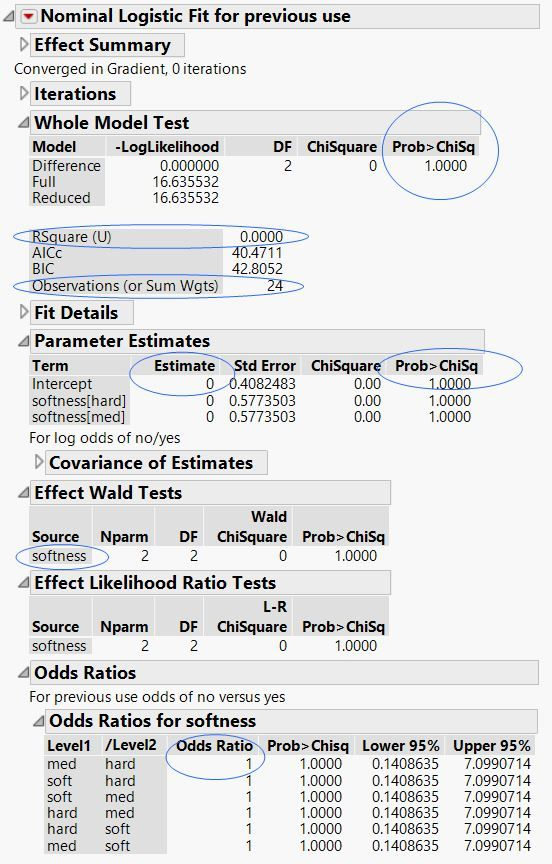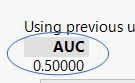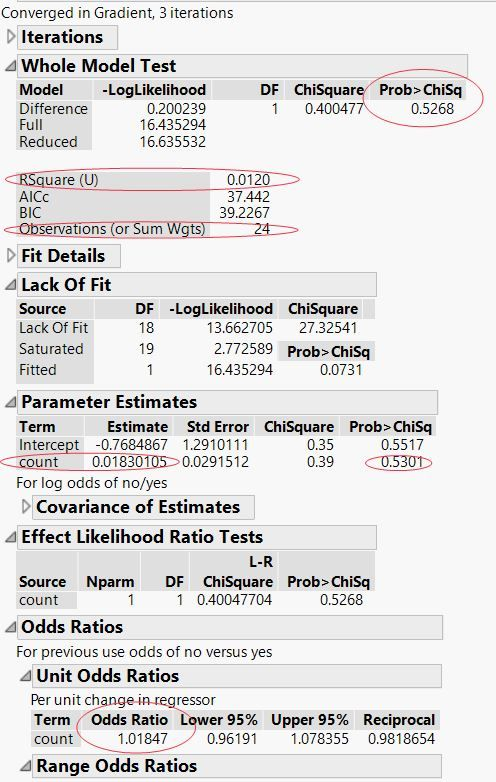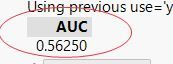Our World Statistics Day conversations have been a great reminder of how much statistics can inform our lives. Do you have an example of how statistics has made a difference in your life? Share your story with the Community!
Choose Language Hide Translation Bar

## Problem

You run an analysis in JMP and want to extract some of the statistics from the platform report. Then you want to place those statistics into a custom report window, so that you can keep those after the data table and platform report are closed.

## Solution

You can get a reference to the report layer of the analysis report and use JSL messages to extract statistics out of

In this example, a Bivariate platform report is generated and you get a reference to the report layer (reportbiv). Using that reference, you can extract variables and strings out of the Bivariate report. Then a new custom report window is created and populated with the statistics and strings that were extracted from the report. Finally, the data table is closed, which closes the Bivariate report, but the custom report window stays open.

``````Names Default To Here(1);

sd = Open( "\$SAMPLE_DATA/Lipid Data.JMP" );

biv = sd << Bivariate( // biv is the analysis layer.
Y( :Triglycerides ),
X( :LDL ),
Density Ellipse( 0.95, {Line Color( {213, 72, 87} )} ),
Fit Line( {Line Color( {57, 177, 67} )} )
);

/* Get a reference to the report layer. */
reportbiv = biv << Report;

// Make sure the "Bivariate Normal Ellipse" outline
// in the Bivariate report is open. You can then see which
// content to extract into the Custom report.
reportbiv[Outline Box( "Bivariate Normal Ellipse P=0.950" )] << Close( 0 );

// Extract the correlation coefficient.
corrvalue = reportbiv[Outline Box( "Bivariate Normal Ellipse P=0.950" )][Number Col Box( "Correlation" )] << Get( 1 );

// Extract the numeric values from the Summary of Fit report as a matrix.
sumfit = reportbiv[Outline Box( "Summary of Fit" )][Number Col Box( 1 )] << Get as Matrix;

// Extract the Term column of the Parameter Estimates report as a list of strings.
term = reportbiv[Outline Box( "Parameter Estimates" )][String Col Box( "Term" )] << Get();

// Extract the Parameter Estimates values as a matrix.
parmests = reportbiv[Outline Box( "Parameter Estimates" )][Table Box( 1 )] << Get as Matrix;

dlg = New Window( "Custom Report",
Outline Box( "Selected Values",
/* The Lineup box defines a two-column layout, each of which contains a Text Box. */
Lineup Box( N Col( 2 ), Text Box( "Factor of Interest: " ), Text Box( term ), ),
tb = Table Box(
/* Display an empty string in the first column and the text in the second column. */
String Col Box( " ", {"Sample Size: ", "Adjusted RSquare: ", "RSquare: ", "Correlation:"} ),
/* Insert a 30 pixel x 30 pixel spacer between the columns. */
Spacer Box( Size( 30, 30 ) ),
/* Display an empty string in the first column and the statistics in the second column. */
Number Col Box( " ", sumfit[{5,2,1}] |/ corrvalue )
),
/* Insert a 1 x 30 spacer. */
Spacer Box( Size( 0, 30 ) ),
Table Box(
/* Display the cloned String Col Box and insert the Parameter Estimates and Standard Error values. */
String Col Box( "Term", term ),
Number Col Box( "Estimate", parmests[0,1] ),
Number Col Box( "Standard Error", parmests[0,2] )
)
)
);

tb << Set Heading Column Borders( 0 ); // Turn off table column borders.
Close( sd ); // Close the data table.
``````

## Discussion

JMP platform reports contain two layers: the platform layer and the report layer. If you have a JSL object that is a reference to the platform layer, you can send messages to that object that relate to the analysis. These are generally options that are found in the red triangle menu. If you have a JSL object that is a reference to the report layer, you can send messages to that object that relate to the report itself. These messages can extract values or images, change selections, open and close outline nodes, among other actions.

I tried to follow this code and use it in a different report.

my intention is to run several models and summarize the important results in a table the way it is shown here.

Here Is a simple example on a sample dataset to show what I’m aiming for.

For starts I tried to extract the P-value of the model. So I commented out the second model and wrote the following code:

``````Open("\$SAMPLE_DATA/Detergent.jmp");

Model1 = Fit Model(
Y( :previous use ),
Effects( :softness ),
Personality( "Nominal Logistic" ),
Run(
Positive Level( "yes" ),
Likelihood Ratio Tests( 1 ),
Wald Tests( 1 ),
Odds Ratios( 1 ),
ROC Curve( 1 )
)
);

reportMod = Model1 << Report;

FMchi = reportMod[Outline Box( "Whole Model Test" )][Number Col Box( "Prob>ChiSq" )] << Get( 1 );

dlg = New Window( "Custom Report11",
Outline Box( "Selected Values",
Table Box(
String Col Box( "Pvalue", FMchi )
)
)
);
/*

Model2=Fit Model(
Y( :previous use ),
Effects( :count ),
Personality( "Nominal Logistic" ),
Run(
Positive Level( "yes" ),
Likelihood Ratio Tests( 1 ),
Wald Tests( 0 ),
Odds Ratios( 1 ),
ROC Curve( 1 ),
Logistic Plot( 1 )
)
);``````

The final table I want is:

 Attribute Whole Model Test RSquare (U) N Estimate Prob>ChiSq Odds Ratio AUC softness 1.000 0.000 24 0 1.000 1.000 0.500 count 0.5268 0.012 24 0.0183 0.5301 1.01847 0.5625

here is a screen shot of the report obtained in which I highlighted the data extracted.

(In attribute softness, for parameter estimate and odds ratio I took only the first row from the journal).The original report window shows up but i didn't even get the additional report window to open.

Any tips would be appriciated.

TIA, Eli

Article Labels

There are no labels assigned to this post.

Article Tags
Contributors